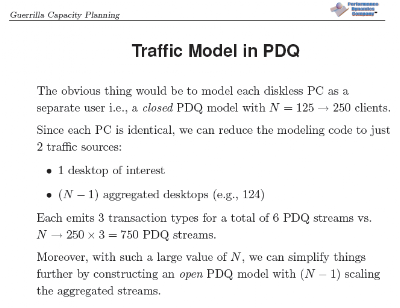## Wednesday, November 18, 2009

### GCaP Class: 3-Tier Queueing Model

Guerrilla Capacity Planning class attendee, Greg S., raised an interesting question during the section where I present a PDQ model of a 3-tier client/server system. The assumptions used to develop the model are summarized on this slide:In the baseline configuration there are 125 desktop clients generating 3 types of database transactions corresponding to 3 different workload classes, or streams in PDQ parlance. This could be represented as either:

1. 125 × 3 workload streams or
2. 1 × 3 streams with 124 × 3 aggregated streams.

The respective arrival rates for the second case look like this:The question is, why is there any difference between the PDQ results produced by representations (1) and (2)?

The distinction is subtle and you could use either approach. In the second case, the PDQ model looks at the impact of the other 124 desktop PCs on the response time of any particular PC. Looking at the Perl::PDQ code, we see that such a perspective introduces a slight asymmetry in the service demands. On the PC of interest, we have the sum of service demands for initiating the request ($CD_Req) and displaying the data returned from the database ($CD_Rpy):
pdq::SetDemand("PC", $txCD,$demand[$CD_Req][$PC] + (5 * $demand[$CD_Rpy][$PC])); but there is no aggregate (background) workload,$bgCD, present on the desktop of interest. Conversely, all 125 PDQ streams do run on the other resources in the client/server network. For example, on the application server (AS):
pdq::SetDemand("AS", $txCD,$demand[$Req_CD][$FS] + (5 * $demand[$CD_Msg][$FS]));pdq::SetDemand("AS",$bgCD, $demand[$Req_CD][$FS] + (5 *$demand[$CD_Msg][$FS]));

In other words, we're evaluating the end-user response time at a given desktop PC in the presence of work generated by the other 124 desktops.